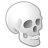# Controling raster precision (pixel type)

2036
0
06-24-2014 10:39 AMNew Contributor II
Hello,

I'm running some raster calculations (spatial analyst, using Python, v10.2.2) and get massive 64-bit double precision rasters
as default output.  Is there any way to force single precision or 32-bit depth aside from this approach:

[INDENT]1. run the calculation e.g.
```r1 = FocalStatistics(inputRaster, nbrValue, statistic, "DATA")
outRas = (r1 / 63.5) ```
where inputRaster is an unsigned 8-bit raster
2. Convert outRas to an integer by multiplying by 1,000, then rounding to keep only the first 3 decimal places
3. Converting back to float (32-bit hopefully) by dividing by 1,000
4. Saving to output format (file GDB or GeoTIFF)
[/INDENT]

Any other way to control pixel type than this?  Thoughts/insights appreciated!

Thanks,
Lisa
Tags (3)
0 Replies